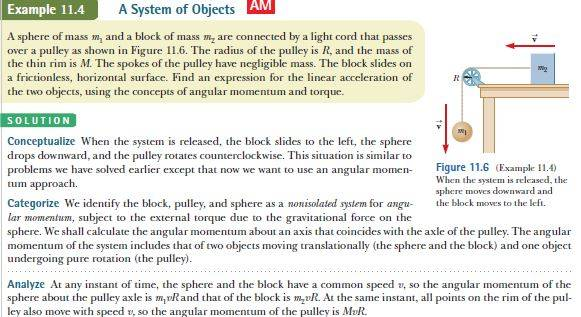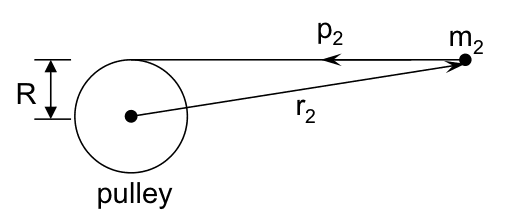# Angular momentum of a pulley system

undividable

## Homework Statement

it is given in the image i uploadedL=r × p[/B]

## The Attempt at a Solution

If the angular momentum of a particle is the cross product of the position vector of the particle from the axis and its linear momentum, how can the angular momentum of m1 and m2 be m1vR and m2vR respectively, R is not the position vector, it's just the radius of the pulley. The position vector should be a vector that starts at the axis and ends at the center of mass of m1 or m2, R is not even equal in magnitude to the position vector of m2 or m1.

## Answers and Replies

Homework Helper
Gold Member
2021 Award
Assume that the entire mass of the sphere and the block is concentrated at one and the other end of the string. Does that help?

Mentor
Have you tried calculating the angular momentum?

undividable
Have you tried calculating the angular momentum?
i have, but the problem says that in the analyzing part it says that m1vR and m2vR are the angular momentum for m1 and m2 respectively, and i can not understand why we use R, that is not the position vector.

undividable
Assume that the entire mass of the sphere and the block is concentrated at one and the other end of the string. Does that help?

i did, and my question remains, why does the text in the image say that m1vR and m2vR are the angular momentum for m1 and m2 respectively, R is not the position vector, even if we consider the center of mass of m1 and m2 as what the position vector is referring to

Mentor
i have, but the problem says that in the analyzing part it says that m1vR and m2vR are the angular momentum for m1 and m2 respectively, and i can not understand why we use R, that is not the position vector.
That tells me you haven't actually calculated ##\vec{L}##. You have masses moving is straight lines, so it shouldn't be complicated to figure out ##\vec{r}## and ##\vec{p}##, and hence ##\vec{L}##.

undividable
That tells me you haven't actually calculated ##\vec{L}##. You have masses moving is straight lines, so it shouldn't be complicated to figure out ##\vec{r}## and ##\vec{p}##, and hence ##\vec{L}##.

well, i cant figure out r

Mentor
well, i cant figure out r
If you choose your coordinate system cleverly, you will find that there is one vector components ##\vec{r}## that is time-dependent, so you don't actually know what it is, but the other two are fixed, and the one you don't know won't matter.

undividable
If you choose your coordinate system cleverly, you will find that there is one vector components ##\vec{r}## that is time-dependent, so you don't actually know what it is, but the other two are fixed, and the one you don't know won't matter.

so the component that is constant is equal to R and is perpendicular to the momentum vector, and the other vector component is time-dependent and parallel to the momentum, so the vector product will equal a vector with components R and 0?•